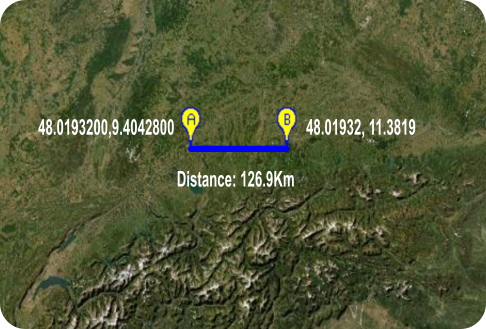# Android TutorialCalculation of Distance in Km between two points on MapFragment

Hi I share a simple solution to calculate the distance in km between two points on MapFragment. I hope it will be of help to anyone who works with the GoogleMaps v2.50 library.

The coordinates used for this example are set in the image.The code is the translation of Haversine's formula.

CODE:
``````Sub Activity_Create(FirstTime As Boolean)

CalcDistance(48.0193200,9.4042800,48.01932, 11.3819)

EndSub

Sub CalcDistance(lat1 As Double,lon1 As Double,lat2 As Double, lon2 As Double)

Dim R As Double=6371

Dim a As Double = ASin(dLat/2)*ASin(dLat/2)+ASin(dLon/2)*ASin(dLon/2)*ACos(alat1)*ACos(alat2)
Dim c As Double=2*ATan2(Sqrt(a),Sqrt(1-a))
Dim d As Double=R*c
Log("Distance: "&Round2(d,1))
Return d

End Sub

Sub toRad(Value As Double) As Double

Dim value2 As Double

value2 = Value*cPI/180

Return value2

End Sub``````

#### WebQuest

##### Active Member
hi emex i'm creating an app that uses the MapFragment and i tested the coordinates in an online simulator that calculates the distance between two points and matches. Perhaps there may be a small difference in decimals.

Last edited:

#### roumei

##### Active Member
I agree with emexes, your calculation seems to be wrong. Here's my code to calculate the distance in meters:
B4X:
``````Public Sub GetSimpleDistanceInMetersBetweenTwoLatLons(lat1 As Double, lon1 As Double, lat2 As Double, lon2 As Double) As Double
Dim Deg2Rad As Double = 2*cPI/360
Dim R As Double = 6371e3
Dim phi1 As Double = lat1 * Deg2Rad
Dim phi2 As Double = lat2 * Deg2Rad
Dim deltaphi As Double = phi2-phi1
Dim deltalambda As Double = (lon2-lon1) * Deg2Rad
Dim sindeltaphihalf As Double = Sin(deltaphi/2)
Dim sindeltalambdahalf As Double = Sin(deltalambda/2)
Dim a As Double = sindeltaphihalf * sindeltaphihalf + Cos(phi1) * Cos(phi2) * sindeltalambdahalf * sindeltalambdahalf
Dim c As Double = 2 * ATan2(Sqrt(a), Sqrt(1-a))
Return R*c
End Sub``````

B4X:
``````Dim SimpleDistance As Double = GetSimpleDistanceInMetersBetweenTwoLatLons(48.0193200,9.4042800,48.01932, 11.3819)
'returns 147083.5 meters``````

•Artur2024 and Erel

#### WebQuest

##### Active Member
Actually trying again now I also notice that there is some error in the calculations I will review the solution•emexes

#### emexes

##### Expert
I will review the solutionSounds great, because it was otherwise a useful snippet. You can just edit the post rather than rewrite it. And while you're busy removing all trace of having human fallibility, I'll do likewise with my posts.•MrKim

Replies
25
Views
3K
Replies
2
Views
2K
Android Question MapFragment problem ?
Replies
6
Views
1K